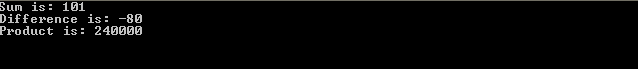Post

# Secrets of Accumulator Functions

In this article, we are going to look into C# feature called as Accumulator functions. In general accumulate means reduce; this functions are so called since it will accumulate/reduce a data structure in some order and build up a return value.

These functions accept three things:

• A combining function
• A Data structure having list of elements and
• A seed/initial value.

It proceeds to combine elements of the list using the function in a systematic way.

Let's see this in an example. Create a new VS 2008 Console application with name as AccumulatorMethodSample. Now, add the below code to Program.cs:

Class Program

{

static void Main(string[] args)

{

int[] myNumbers = { 10, 20, 30, 40 };

int mySum = myNumbers.Aggregate(1, (s, n) => s + n);

int myProduct = myNumbers.Aggregate((s, n) => s * n);

int myDifference = myNumbers.Aggregate((s, n) => s - n);

Console.WriteLine("Sum is: "+mySum.ToString());

Console.WriteLine("Difference is: "+myDifference.ToString());

Console.WriteLine("Product is: "+myProduct.ToString());

}

}

In above code, Aggregate accumulator is accepting seed value as 1 and a lambda expression saying to add the values. This function is getting a value by accumulating the myNumbers list, without modifying it.

For all the accumulators, there is default seed value based on the operation being performed:

 Operation Default Seed + 0 - 0 * 1 / 1

Run the application, the output will be like this:Now, let's see accumulator internal calculation for above operations:

For + [addition], the value will be calculated in below way:
((1 + 10) + 20 ) + 30 ) + 40 ) = 101

For - , the value will be calculated in below way:
(((10 – 20) – 30) – 40) = -80

In this way, it works by taking the accumulator and the first element in the list and applies the lambda expression on them. The result becomes a new accumulator. Then the expression is applied on the new accumulator and the second element of the list. This process is repeated with all the elements of the list. Built-in accumulator functions are available over all the collections as an Extension method present in System.Linq namespace. Now, we will move over creating our own accumulator.

Modify the Main method with below code:

static void Main(string[] args)

{

int[] myNumbers = { 10, 20, 30, 40 };

int mySum = myNumbers.Aggregate(1, (s, n) => s + n);

int myProduct = myNumbers.Aggregate((s, n) => s * n);

int myDifference = myNumbers.Aggregate(1,(s, n) => s - n);

int myLeastNbr =Accumulator((s, n) => (s >= n) ? n : s, Int32.MaxValue, myNumbers);

Console.WriteLine("Sum is: "+mySum.ToString());           Console.WriteLine("Difference is: " + myDifference.ToString());

Console.WriteLine("Product is: "+myProduct.ToString());

Console.WriteLine("Least Number is: " + myLeastNbr.ToString());

}

public delegate T Func<T>(T val1, T val2);

public static T Accumulator<T>(Func<T> function, T accumulator, IEnumerable<T> list)

{

foreach (T listItem in list)

accumulator = function(listItem, accumulator);

return accumulator;

}

}

Here, we are defining a delegate, which will act as a placeholder for function's behavior as a first argument in Accumulator function. Finally, we are defining our own Accumulator with a recursive call to the lambda expression with a new accumulator's value in for loop. Once the elements in the list are completed, we are returning the final result.

Run the application, the output will be like this:In this way, we can write our own accumulator functions. These functions are also called as Fold functions.

I am ending the things here. I am attaching source code for reference. I hope this article will be helpful for all.

Recommended Free Ebook
Similar Articles
"